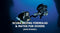## Scuba Diving Formulas & Maths For Divers

Maths is an absolute science and there are a few sums that will help you as a diver.

• How much air do you have in your cylinder?
• How long will my air last?
• How much air do I need to have a third left at the end of the dive?

Here are a few sums to allow you to answer all of these questions, so let's show you how to work them all out.

#### How much air do you have in your cylinder?

If you have a 12 litre cylinder filled to 200 bar you have 2,400 litres of air. While a 15 litre cylinder filled to 220 bar has 3,300 litres and twin 12’s filled to 210 bar have 5,040 litres. A simple sum, cylinder size in litres, multiplied by the pressure in bar. 12 X 200 = 2,400 litres 15 X 220 = 3,300 litres 12 X 2 = 24 X 210 = 5,040 litres So now you can work out the following: How many litres in of air in a dumpy 12 filled to 190 bar? Your cylinder is filled to 210 bar and it is a steel 15 litre?

#### How long will my air last?

This sum is a bit more complicated as we have to know how much air we use before we can work out how long it will last. You may have heard people talking about their SAC rate. What they mean is how much air would they be using at the surface or their Surface Air Consumption (SAC) rate. We all learnt in our open water training that the deeper we are diving the more air we use so we use the surface rate as the control rate.

First, to find out our SAC rate, the simple way is to spend several hundred pounds on an intergrated air dive computer and laptop. This way, the computer does it for you. Or you could dive to a simple depth like 10 metres and note the pressure in your cylinder. Then swim around at 10 meters for ten minutes and check your pressure again. You now know how much air in bar that you use at 10 metres, in ten minutes. So if your start pressure was 190 bar and your finish was 170 bar you have used 20 bar. Remember that we need to know the size of your cylinder, as we learnt in the last sum, that size matters!

#### Litres per minute

To find your SAC rate in litres per minute you need to complete four sums. First multiply the bar used by the cylinder size. So, 20 bar multiplied by 12 litres, equals 240 litres. Second, we work out a depth value by taking the depth, adding 10, and dividing that number by 10. So, 10 metres + 10 = 20, divide by 10, equals 2. Thirdly, we then divide the first number by the second. So, 240 divide by 2 equals 120. Finally we divide this number (120) by the time in minutes (10). So, 120 divide by 10, equals 12 litres per minute Litres per minute = bar used X cylinder capacity litres divide by (depth+10)/10, divided by minutes. Once more then, at 20 metres we use 40 bar, in 10 minutes whilst using in a 15 litre cylinder. 40 X 15, divide by 20 + 10 divide by 10, divided by 10. 40 X 15 = 600 20 + 10 = 30 divide by 10 = 3 600 / 3 = 200 200 / 10 = 20 litres per minute.

#### How long will my cylinder last?

So, now you know how to work out your SAC rate you can calculate how long the cylinder will last. If we use 20 litres a minute at the surface, a 15 litre cylinder containing 200 bar would last 150 minutes (15 X 200 / 20). Not a lot of use when diving as we do not want to spend the time on the surface! So, if we want to complete a 45 minute dive at 17 metres, how do we know if we have enough air? Gas needed equals (time in mins X SAC rate) X ((depth+10)/10) 45mins x 20li/m = 900li, multiplied (17metres + 10) 27/10 = 2.7 This means that you will need 2,430 litres of air to complete the dive.

#### How much air do I need to have a third left at the end of the dive?

We know from the first sum that a 12 litre cylinder will not be big enough and if you apply the rule of thirds you need to have an extra third of air left at the end of the dive. There is a simple sum to work this out, multiply the air needed by 1.5 so to complete the dive you will need to multiply 2,430 by 1.5, which means you need 3,645 litres. Unless you dive side mount or twins you will need to reduce the dive time or reduce your SAC rate. If you want or need more air for your dives have a look at the links below and speak with the guys at Scuba Leeds about Twinset or Sidemount training! Sidemount Discover Sidemount Tec40 Discover Twinset
Dive school